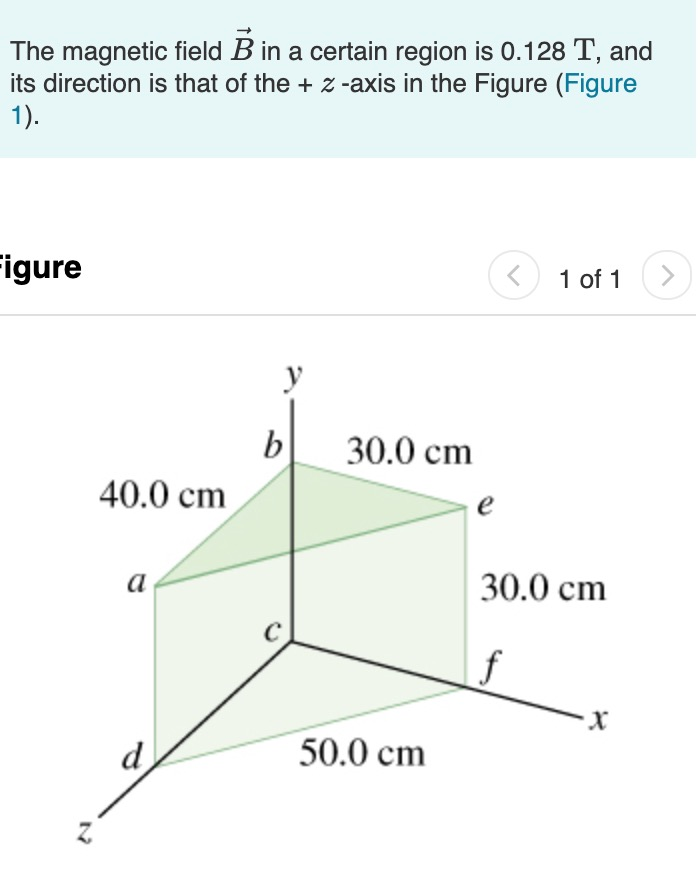# Problem: Part A. What is the magnetic flux across the surface abcd in the figure?Part B. What is the magnetic flux across the surface befc?Part C. What is the magnetic flux across the surface aefd?Part D. What is the net flux through all five surfaces that enclose the shaded volume?

###### FREE Expert Solution

Magnetic flux:

$\overline{){{\mathbf{\varphi }}}_{{\mathbf{B}}}{\mathbf{=}}{\mathbf{B}}{\mathbf{·}}{\mathbf{A}}{\mathbf{·}}{\mathbf{c}}{\mathbf{o}}{\mathbf{s}}{\mathbf{\theta }}}$

Part A

Area abcd = 0.4 × 0.3 = 0.12 m2

The z-axis and normal to area abcd are perpendicular.

ϕB = BAcosθ = (0.128)(0.12)(cos90°) = 0 Wb

85% (361 ratings)###### Problem Details

Part A. What is the magnetic flux across the surface abcd in the figure?
Part B. What is the magnetic flux across the surface befc?
Part C. What is the magnetic flux across the surface aefd?
Part D. What is the net flux through all five surfaces that enclose the shaded volume?